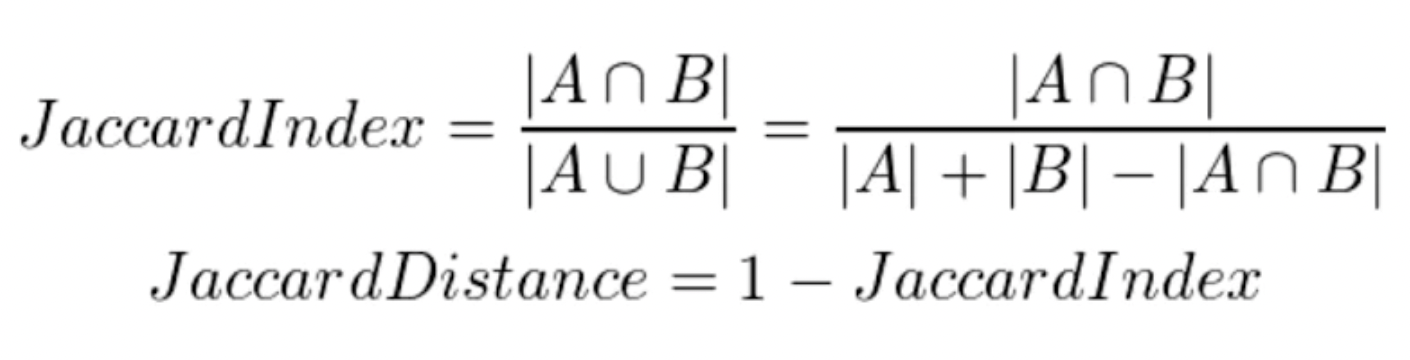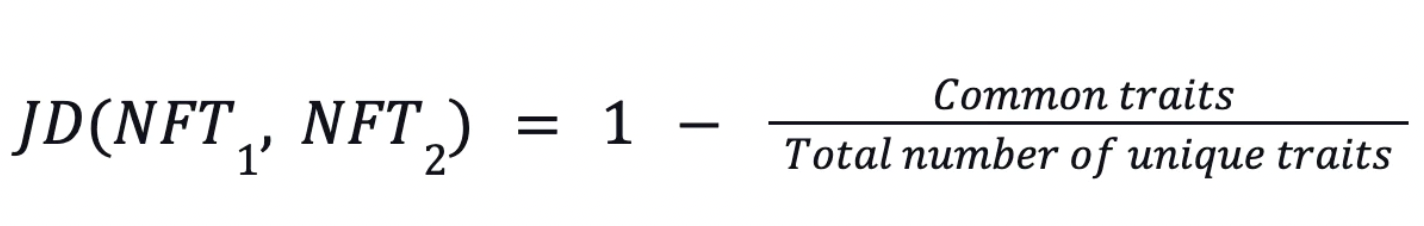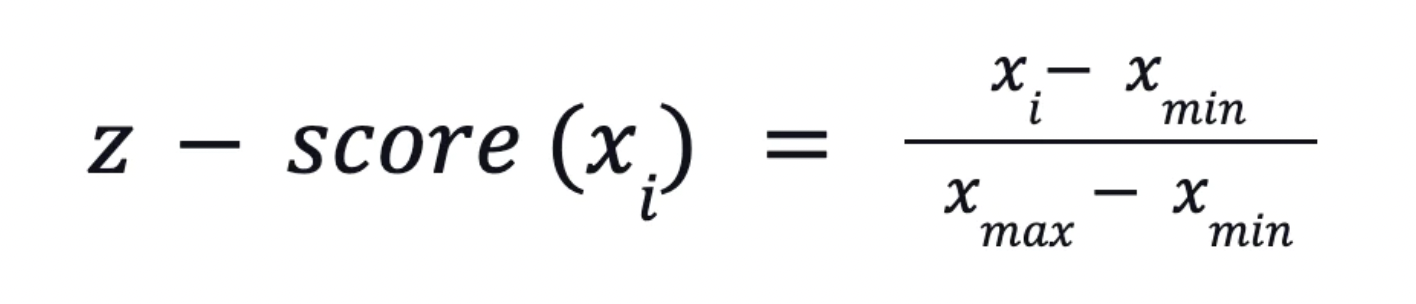# GoRarity

A Rarity Model Self-developed by NFTGo.io

### Introducing GoRarity

#### How is Rarity Determined?

The rarity of an object is mainly dependent on its differences from other objects. The greater the difference, the more special this object will be. Therefore, quantifying the difference between an object and other objects within the same group will reflect the object's rarity.

#### What is Lacking with Mainstream Rarity Determination Methods?

Mainstream rarity determination methods often need to be revised and more accurate. This is evident in the market, with certain apes ranking high in terms of rarity despite not having any unique attributes.

This problem arises as existing models naively add up the traits and frequency of occurrence for each trait. However, this simple approach must consider how each trait contributes to a particular NFT's uniqueness.

Based on the above logic, NFTGo.io uses a statistical approach known as Jaccard Distance to evaluate the actual rarity of NFTs. This innovative NFT rarity evaluation goes beyond the mainstream approaches employed by other platforms and takes a more scientific approach to determine NFT's rarity.

With this higher accuracy rarity model, users will be able to identify mispriced NFTs on the market, meaning that the price is low despite having a relatively higher rarity. This will give users a huge edge over the broader market and allow them to buy such NFTs. Once the market picks up on the actual rarity of the NFT, demand will surge, resulting in a price increase, allowing users to profit from it.

### What is Jaccard Distance?

A metric is used to measure the similarity between 2 sets of data. With NFTGo.io's rarity model, the Jaccard Distance considers the similarities between NFTs based on their attributes. A rarity score ranging from 0 to 100 is then assigned to the NFTs. With 0 representing the least rare and 100 representing the rarest.

With this algorithm employed, NFTGo.io's rarity model can quantitatively measure the similarities between 2 NFTs. This opens the possibility of estimating an NFT's rarity scientifically and more accurately.

### Jaccard Distance Formula

Firstly, the Jaccard Index is calculated. This refers to the distance between 2 data points. In the case of NFTGo.io's rarity model, this is calculated by taking the number of similar attributes divided by the total number of attributes between the 2 data points.

Jaccard Distance, the final rarity indicator, is then calculated by subtracting the Jaccard Index from 1.### Deeper Dive into Jaccard Distance

Jaccard Distance measures the overlap between 2 finite sets. Jaccard Distance is inversely related to the magnitude of overlap, where the higher the overlap, the lower the Jaccard Distance. Through understanding the similarities across NFTs within a collection, the rarity of a NFT can be gauged. Every NFT in the collection is compared to all other NFTs. A Jaccard Distance will be calculated for each pair, and the results for the whole collection will be normalized to get the optimal rarity.

There are 4 main steps in calculating an NFT's rarity.

#### Step 1

The number of similar traits between the 2 NFTs is divided by the total number of unique attributes. This number obtained is subtracted from 1. This is repeated for all NFT pairs within the collection.#### Step 2

The average of the results from step 1 is obtained using the formula below.#### Step 3

The result obtained from step 2 is normalized to obtain the z-score.#### Step 4

Finally, the z-score is multiplied by 100 to obtain a number within the range of 0 to 100, which will be the final rarity score of the NFT.

More information pertaining to NFTGo.io's built in-house rarity model can be found here.